# On necessary precautions when measuring solid polymer linear viscoelasticity with torsion rheometers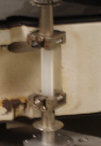The linear viscoelasticity and time-temperature superposition of solid polymers are often characterized using a torsion rheometer since it is a common equipment in polymer labs. Specimen are submitted to a dynamic torsion angle while the resulting torque is recorded. The torsion test gives access to the storage and loss shear moduli. When rectangular slender specimen are tested, the Saint-Venant solution calculated for free-end torsion of prisms is used to express the storage modulus in terms of the applied torsion angle amplitude θ_0 and the resulting torque amplitude M_0 :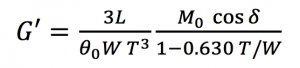with LxWxT, the length, width and thickness of the sample.

In order to illustrate the erroneous results that may be obtained when tests are run without care, we are considering a mere acrylate polymer network. First, two rectangular specimens of different geometries, length x width x thickness being 20x12x1 mm3 and 46x5x1 mm3, were submitted to classic temperature sweeps at 1 °C/min at constant frequency 1 Hz and constant strain amplitude 0.1%. Figure 1 shows the values of the storage modulus and damping factor for both samples. One notices that results depend rather strongly of the specimen geometries. In order to prove that the relatively slow temperature ramp 1 °C/min is not necessarily slow enough to ensure homogeneous temperature through the whole sample, dynamic tests were run at given stabilized temperatures.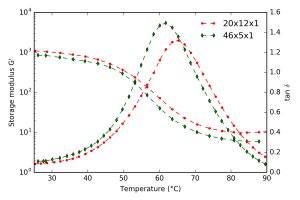Figure 1. Impact of specimen geometry on the storage modulus and daming factor measured during a ramp temperature at 1 °C/min at 1 Hz and 0.1% strain. Specimen dimensions are given in length x width x thickness.

Figure 2 presents, the values of storage modulus and damping factor obtained for both samples at given temperatures that were stabilized before running the dynamic test at 1 Hz and 0.1% strain. One notices that the damping factor values coincide for both geometries, while the storage moduli are still different.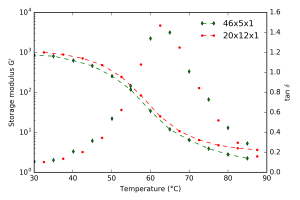Figure 2. Storage modulus and damping factor at various stabilized temperatures for dynamic tests at 1 Hz and 0.1% strain.

In order to understand the discrepancy between the storage modulus values, we run finite element simulations of the torsion tests for the different geometries considered here, assuming the material as linear viscoelastic. When comparing the mises stress within the samples (Figure 3), we may see that for short samples, Saint-Venant solution is not a satisfactory approximation anymore due to the clamping disruption. Therefore, when possible tests should be run on specimen with high aspect ratios L/W and W/T.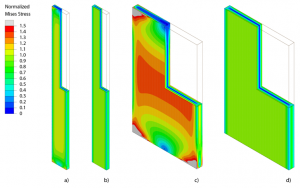Figure 3. von Mises stress within samples submitted to torsion along the vertical axis. The vertical displacements in the upper and lower section is free (like for Saint-Venant torsion prism solution) in b) and d) and is prevented (like when clamps are used during actual tests) in a) and c).

The values of storage modulus obtained with the approximated Saint-Venant solution should be divided by a factor K depending on the specimen shape factors and the material Poisson’s ratio in order to reach the actual storage modulus values. In order to calculate this parameter, various finite element simulations with varying geometry shape factors and Poisson’s ratio values were run. The following expression of K was obtained,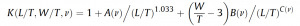For Apple users, an app was written calculating the value of K when L,W,T and the Poisson ratio are given; ask at julie.diani [] gmail.com.

If interested, the paper J. Diani, P. Gilormini, 2017. On necessary precautions when measuring solid polymer linear viscoelasticity with dynamic analysis in torsion. Polymer Testing, 63, 275-280 may be downloaded there.